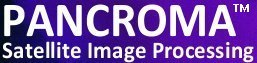See additional satellite image processing articles at www.PANCROMA.com menu selection 'White Papers'

# Landsat Spectral Unmixing

 Spectral unmixing represents an interesting approach to understanding and using multispectral data. The method starts with an assumption that the characteristics of observed image pixels constitute mixtures of the characteristics of a small number of basic cover types, called endmembers. If the mixtures are assumed to consist of linear combinations of the contributions of the individual cover types, a linear mixing model can be formulated. This linear mixing model can be used to determine the fractions of each cover type present in the image. For example, consider the six Landsat visible plus NIR bands. A reflectance value can be computed for each pixel in the image, a different (usually) reflectance for each band corresponding to that pixel. Let's assume that these reflectances, measured by the satellite sensors are completely the result of reflectances from four cover types. As a result, each cover type will contribute a fraction F (referred to as endmember Abundance) of its individual reflectance X at that wavelength to the measured reflectance B for each multispectral Landsat band. Let's further assume that we know the reflectances X in each multispectral wavelength for each of the four end members. These can be obtained from libraries as described in previous sections, or from actual ground measurements. F and B can be considered column vectors, F composed of the four unknown Abundances and B composed of the six reflectances (one for each multispectral band) measured by the satellite. We can write the linear relationship among X, F and B as an equation as follows: X00 * F0 + X01 * F1 + X02 * F2 + X03 * F3 = B0 This is just the standard linear mixing equation. We can formulate a set of linear equations that relate the reflectances in each wavelength of the end members to the measured reflectances Y. The relationship is shown in matrix form below.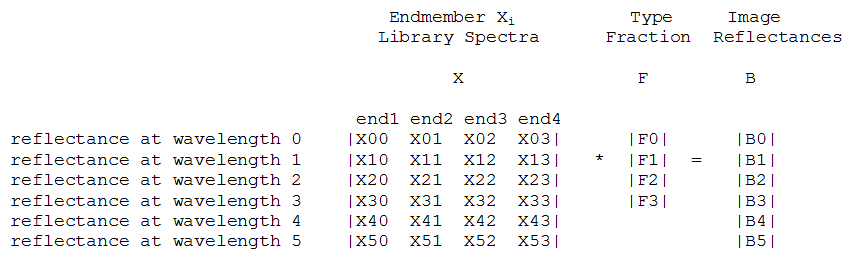Matrix Expression Note that if we had only four equations we could solve for the fractions F exactly. We can get a better solution by including all of the multispectral data we have, and then solving the set of over determined equations using linear regression. Note that the system of equations that we have to solve applies to one pixel only. We will have to repeat the solution process for every pixel in the image. This is obviously a computationally expensive task but it can be accomplished by the PANCROMA™ regression solver. The solution yields four sets of fractions F, one for each pixel in the image. These fractions are usually depicted graphically by scaling them so that the range from 0 to 255 and then rendering them as four grayscale images. The higher (whiter) the grayscale pixel value, the greater the fraction of that particular end member in the pixel. This process can be illustrated with an example. Consider the Landsat band1 image below.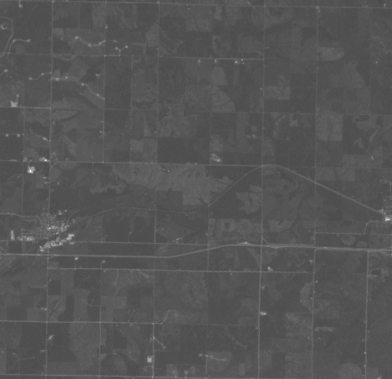Landsat Band 1 Image For this example, lets assume that the reflectances measured by the satellite are the result of reflectances from just four end members: cheatgrass, asphalt, dry grass and fresh oak leaves. We can obtain reflectance spectra for each of these from the USGS Spectral libraries. Start by opening each of the six Landsat multispectral bands, that is bands 1,2,3,4,5 and 7 (recall that band 6 is the thermal infrared band) by selecting 'File' | 'Open' and selecting each of the six band files in succession. Now select 'Spectral Analysis' | 'Landsat Spectral Unmixing' | 'Four End Members'. The files will be read and the Spectral Unmixing form will appear. Referring to the nominal multispectral band wavelengths and the USGS spectral data, enter the reflectances for each end member at each of the six Landsat wavelengths. The nominal wavelengths are shown in the table below.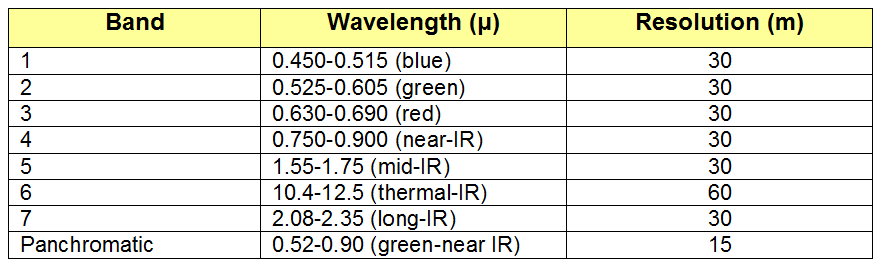Landsat Sensor Characteristics The table with the manually input reflectance values for my selected endmembers is shown below. (Reflecatances were obtained from the USGS Spectral Library tables).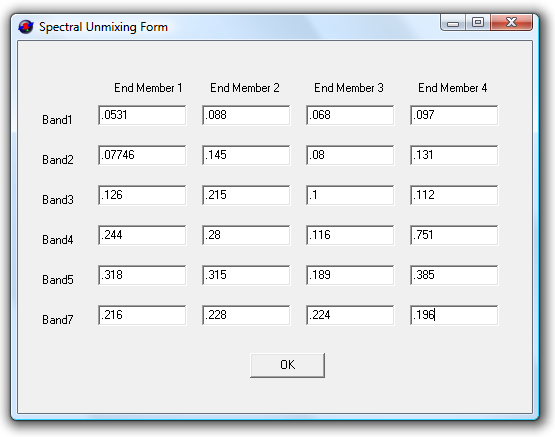Endmember Spectra Select 'OK' and the computation will start. Because a linear regression computation must be run for each pixel in the image, run times may take some time, i.e. ten or fifteen minutes for a full Landsat scene. System RAM will be heavily used as well, so it is wise to shut down other applications during a four-endmember run. The output images from my run are shown below. Theoretically, these show the relative abundances of each of the four cover types corresponding to the four spectra that were input to the model.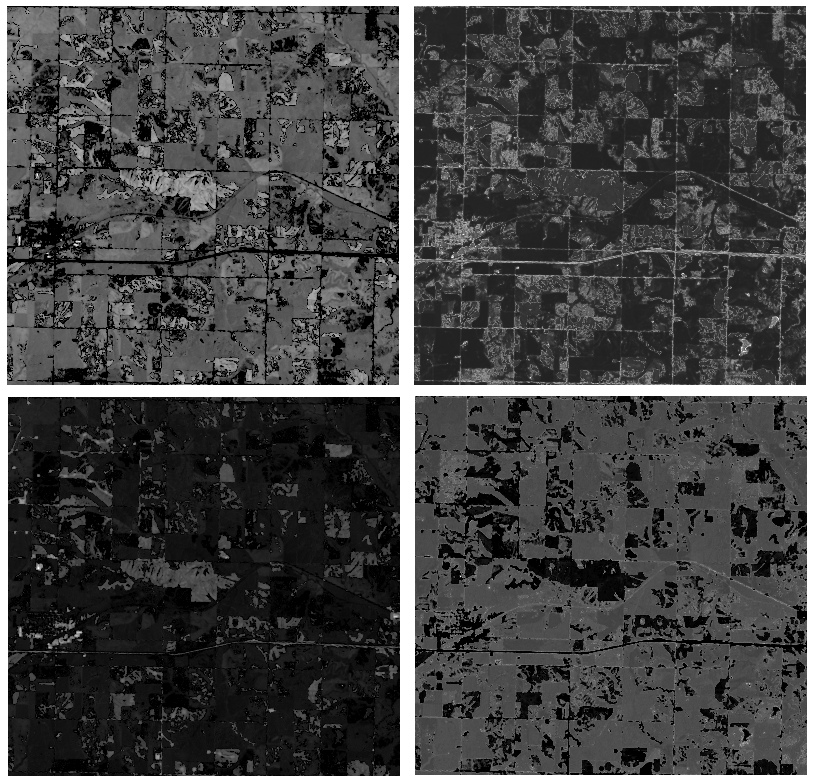Endmember Abundances PANCROMA™ currently allows three, four and five endmember analyses for Landsat data. (Note that five-endmember analysis requires disk scratch files so certain parts of the computation may take considerably longer as stored data is recovered from disk). There may be more or less cover types in a particular image and all of the types may not be identified. Future releases will allow a greater range of endmember types but identification of the endmembers will still be an issue. As with any multispectral analysis method the model may not always match reality and there are lots of provisos to consider for the method. The most obvious is how to determine the number and type of endmembers in the scene. Also, In order to get good results, the library spectra must be calibrated to the satellite sensor. This can be done by taking ground readings with a spectrophotometer or other means. It is perfectly legitimate to compute negative abundances from a mathematical standpoint. This can sometimes occur for a variety of reasons. Unfortunately this does not correspond to physical reality. If negative abundances are computed, PANCROMA™ will substitute a zero for the negative value in the endmember image. The number of substitutions is counted and reported to the dialog screen. A large number of negative abundances would cast doubt on the validity of the analysis. Spectral unmixing provides another interesting approach to multispectral analysis. This is an area in need of more improvement but with a lot of potential. Watch for additional PANCROMA™ capabilities in this area.